Browse by Topic

# New in 13.1: College and Fractional Calculus

Last year we released Version 13.0 of the Wolfram Language. Here are the updates in college and fractional calculus since then, including the latest features in 13.1.

### College Calculus

Transforming college calculus was one of the early achievements of Mathematica. But even now we’re continuing to add functionality to make college calculus ever easier and smoother to do—and more immediately connectable to applications. We’ve always had the function D for taking derivatives at a point. Now in Version 13.1 we’re adding ImplicitD for finding implicit derivatives.

So, for example, it can find the derivative of xy with respect to x, with y determined implicit by the constraint x2 + y2 = 1: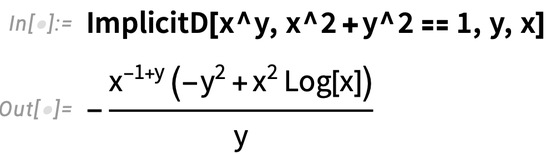✕

Leave out the first argument and you’ll get the standard college calculus “find the slope of the tangent line to a curve”: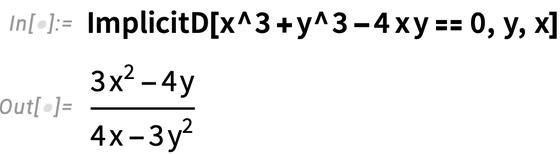✕

So far all of this is a fairly straightforward repackaging of our longstanding calculus functionality. And indeed these kinds of implicit derivatives have been available for a long time in Wolfram|Alpha. But for Mathematica and the Wolfram Language we want everything to be as general as possible—and to support the kinds of things that show up in differential geometry, and in things like asymptotics and validation of implicit solutions to differential equations. So in addition to ordinary college-level calculus, ImplicitD can do things like finding a second implicit derivative on a curve defined by the intersection of two surfaces: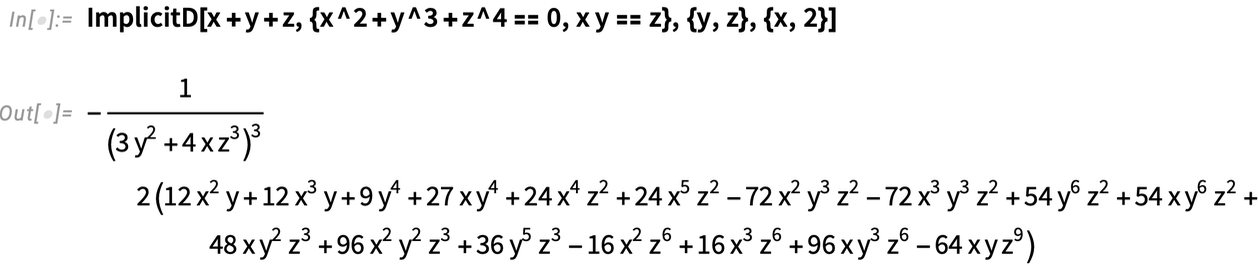✕

In Mathematica and the Wolfram Language Integrate is a function that just gets you answers. (In Wolfram|Alpha you can ask for a step-by-step solution too.) But particularly for educational purposes—and sometimes also when pushing boundaries of what’s possible—it can be useful to do integrals in steps. And so in Version 13.1 we’ve added the function IntegrateChangeVariables for changing variables in integrals.

An immediate issue is that when you specify an integral with Integrate[...], Integrate will just go ahead and do the integral: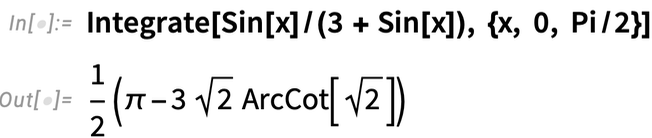✕

But for IntegrateChangeVariables you need an “undone” integral. And you can get this using Inactive, as in: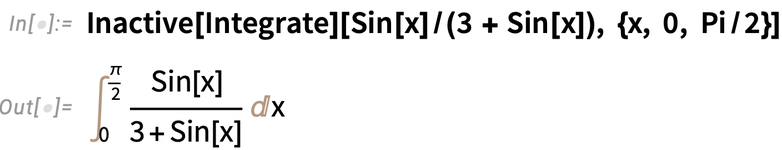✕

And given this inactive form, we can use IntegrateChangeVariables to do a “trig substitution”: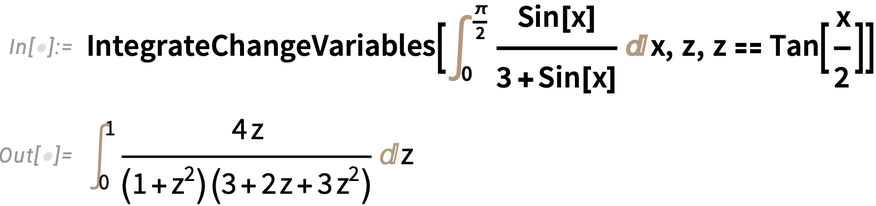✕

The result is again an inactive form, now stating the integral differently. Activate goes ahead and actually does the integral: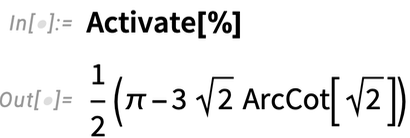✕

IntegrateChangeVariables can deal with multiple integrals as well—and with named coordinate systems. Here it’s transforming a double integral to polar coordinates: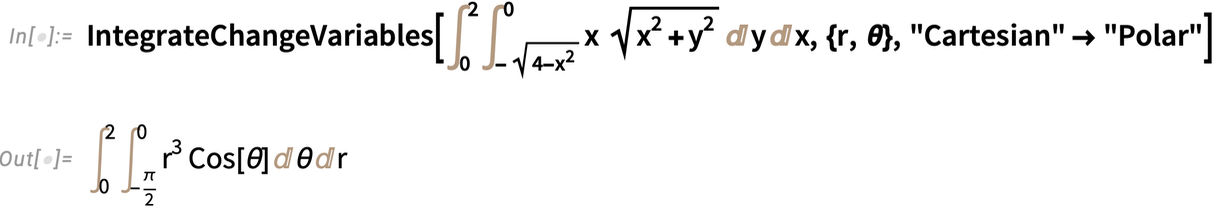✕

Although the basic “structural” transformation of variables in integrals is quite straightforward, the whole story of IntegrateChangeVariables is considerably more complicated. “College-level” changes of variables are usually carefully arranged to come out easily. But in the more general case, IntegrateChangeVariables ends up having to do nontrivial transformations of geometric regions, difficult simplifications of integrands subject to certain constraints, and so on.

In addition to changing variables in integrals, Version 13.1 also introduces DSolveChangeVariables for changing variables in differential equations. Here it’s transforming the Laplace equation to polar coordinates: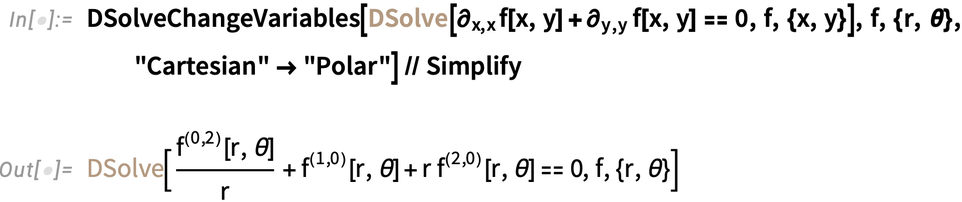✕

Sometimes a change of variables can just be a convenience. But sometimes (think General Relativity) it can lead one to a whole different view of a system. Here, for example, an exponential transformation converts the usual Cauchy–Euler equation to a form with constant coefficients: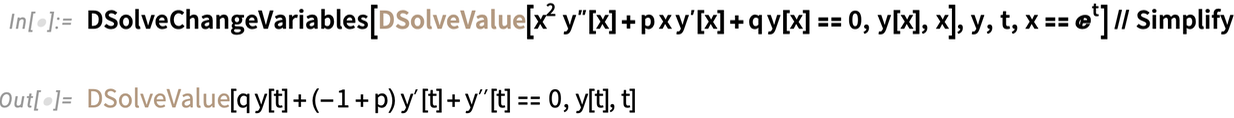✕

### Fractional Calculus

The first derivative of x2 is 2x; the second derivative is 2. But what is the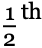derivative? It’s a question that was asked (for example by Leibniz) even in the first years of calculus. And by the 1800s Riemann and Liouville had given an answer—which in Version 13.1 can now be computed by the new FractionalD: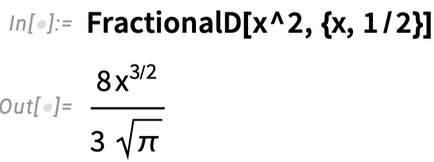✕

And, yes, do anotherderivative and you get back the 1st derivative: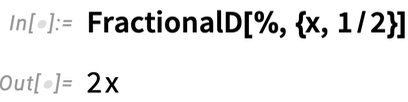✕

In the more general case we have: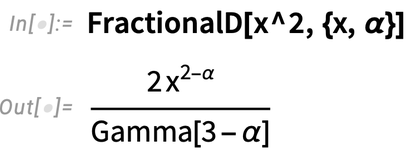✕

And this works even for negative derivatives, so that, for example, the (–1)st derivative is an ordinary integral: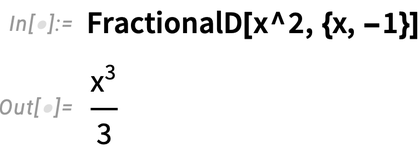✕

It can be at least as difficult to compute a fractional derivative as an integral. But FractionalD can still often do it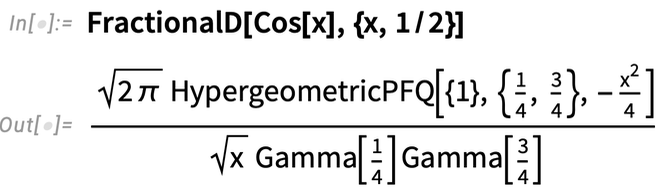✕

though the result can quickly become quite complicated: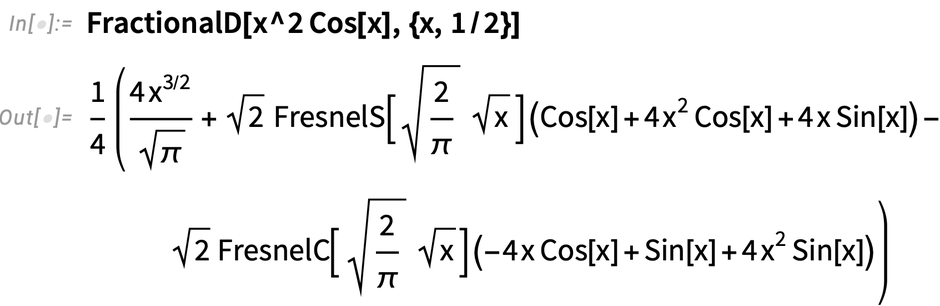✕

Why is FractionalD a separate function, rather than just being part of a generalization of D? We discussed this for quite a while. And the reason we introduced the explicit FractionalD is that there isn’t a unique definition of fractional derivatives. In fact, in Version 13.1 we also support the Caputo fractional derivative (or differintegral) CaputoD.

For the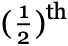derivative of x2, the answer is still the same: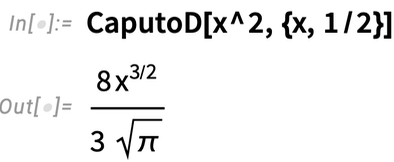✕

But as soon as a function isn’t zero at x = 0 the answer can be different: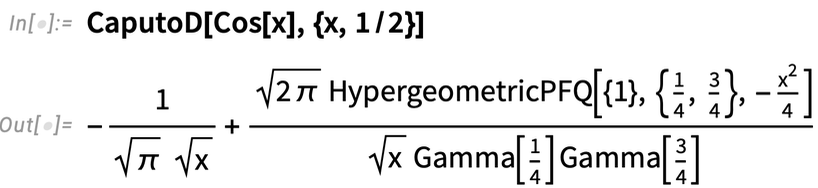✕

CaputoD is a particularly convenient definition of fractional differentiation when one’s dealing with Laplace transforms and differential equations. And in Version 13.1 we can now only compute CaputoD but also do integral transforms and solve equations that involve it.

Here’s a-order differential equation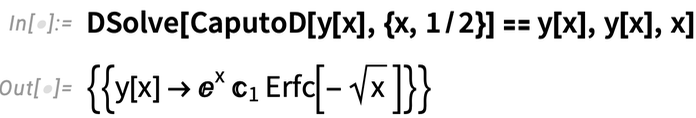✕

and a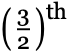-order one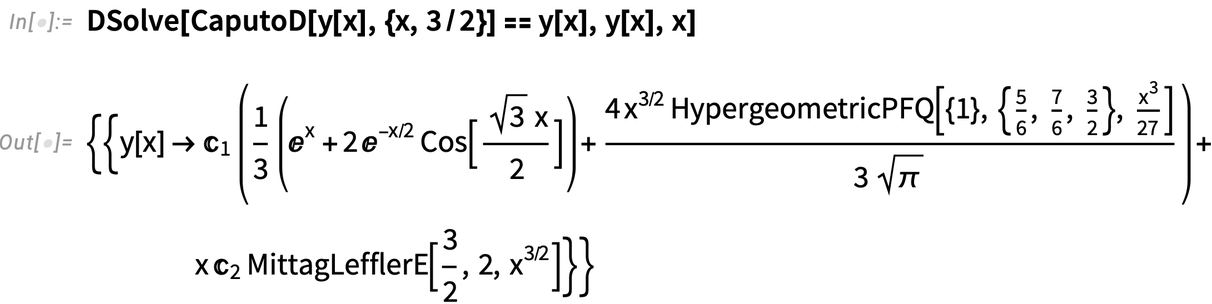✕

as well as a πth-order one: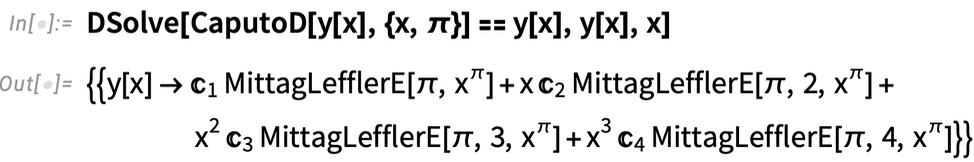✕

Note the appearance of MittagLefflerE. This function (which we introduced in Version 9.0) plays the same kind of role for fractional derivatives that Exp plays for ordinary derivatives.